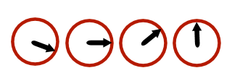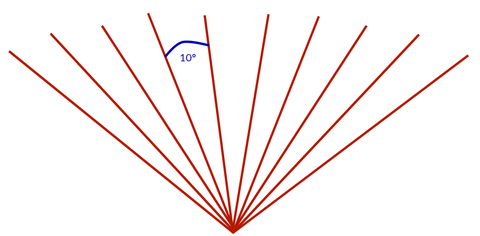# Understanding resolution in mmWave Sensors

mmWave Radar systems work by sending out a “chirp” into space and “listening” for a response. There are lots of parameters that can be tuned with Radar that affect the resolution, and they all come with trade-offs. To increase something means decreasing something else. The configuration needs to be tuned for a specific application.

There are three types of resolution that are important with mmWave Radar. Distance (range) resolution, velocity resolution and angular resolution. Each of these has some unique characteristics which are not as intuitive as they are in other sensing domains. Each of these resolutions is configurable to some extent, but there are always trade-offs. The purpose of this article is to demystify how to think about each of the different resolutions and what trade-offs are made with each.

## Range Resolution

Range resolution is probably the easiest to understand. Radar works by sending out short pulses “chips” and listening for the reflections back. The time it takes between the sending and receiving can be measured, and from this, the distance can be inferred (distance = velocity [speed of light] x time). Radar can detect multiple objects at once; This is achieved by listening for multiple reflections.

The way the receiving of signals works is key to understanding range resolution. The component responsible for receiving data is known as an Analog to Digital Converter (A2D). Its job is to take an analogy signal and convert it into 0’s and 1’s. A2D’s have a sample rate they run at, and this dictates the size of each “bin” they record data into. From a radar perspective, the bin size equates to a fixed distance, and it is this distance that dictates the distance resolution. For radar, the A2D speed dictates the distance resolution that can be achieved.Increasing the sample rate will have the effect of decreasing the bin size and increasing the distance resolution. There is a trade-off to this however. Like all computer systems, there is a fixed amount of memory available. If for example, there were 256 “bins” available for storing data. increasing the sample rate would lower the maximum range because the number of bins would run out faster. On the other hand, slowing down the sample rate will increase the bin size and increase the maximum range, while simultaneously decreasing the range resolution.

Sometimes distance resolution is referred to as the “maximum distance between objects”. This is because if two objects are closer than this distance, they will both appear in the same “range bin”. For FMCW radar that can see 10m, this bin size is typically about 40mm.

But isn’t mmWave Radar supposed to measure distances to sub-millimetre accuracy? The answer is yes. This is achieved by interpolation between range bins and there are several techniques for doing this. It is even possible to measure very small distance changes even more accurately using more advanced techniques.

Range resolution is more accurately described as the “maximum distance between objects”. The “raw” range resolution is dependent on the range bin size, which is in turn dependent on the A2D sample rate.

## Velocity resolution

Velocity resolution is the resolution at which the velocity of an object can be determined. Velocity information is understood from differences in the phase angle of adjacent radar chirps. The math of how this works gets a bit heavy and is beyond the scope of this article, but, there is a maximum velocity that the radar can measure for a particular configuration. If an object moves faster than this limit, the radar will estimate the velocity as much less.

This limit is configurable however, but there are trade-offs. To increase the maximum velocity, chirps must be spaced farther apart, but if chirps are spaced farther apart then there is less data collected overall in a fixed period of time and less data means a lower velocity resolution. So maximum velocity and velocity resolution are inversely related to each other.### Angular Resolution

Angular resolution is the third key dimension where resolution is important. Angular resolution is the ability of the radar to resolve objects in the azimuth direction. mmWave Radar typically has an angular resolution of several degrees. While Radar is great at distance and speed, determining the angle of an object relative to the radar is not possible with the same resolution.

The angular resolution is calculated from the number of “virtual antennas” aligned on the axis of interest and their spacing. The calculation and physics behind this is quite complicated but as a general rule, the angular resolution is around 10 degrees for a 3Tx 4Rx Radar. The actual angle of an object can be interpolated in a similar way as distance.

The angular resolution of radar cannot be configured as it can with distance and velocity because it is related to the physical geometry of the antenna.## Conclusion

In summary, there are three types of resolution that are important to understand for radar. Each resolution comes with a set of trade-offs that must be weighed up and optimised based on the application being designed for.